# Ohms Law – Heat and Power

Every electronic and electrical appliance in this world runs with power. When this happens, some energy is dissipated in the form of Heat. Whereas the Power is the flow of voltage and current in a conductor or semiconductor.

As you know conductor is a material that allows the flow of electrons when an electric field (E.M.F.) is applied. Electrons in pure conductor like copper move easily due to their less resistance.

But, electrons in a conductor such as constantan are less influenced by an external force. They need more driving force to conduct. This extra energy makes the conductor warm and hence the temperature increases drastically.

To understand heat, here is a simple example. If we do some work (running or walking) for a long time, we become warm and get tired.

In electricity also, the same thing happens. Heat is generated by electric current. For instance, inside an electric heater, a soldering iron (soldering bit) is used.

The soldering iron contains a chrome-nickel wire tied to an insulating former. The wounded resistance element is fixed to a copper rod. When the electric heater is turned on the wire gets heats up.

Now let’s take up a little experiment to verify ohms law relation with power. I will demonstrate 3 cases proving the importance of voltage, current, and power.

To do this experiment we need a torch bulb, current meter, and 4V Battery source.

#### Case 1:  One Bulb, Single Battery, and an Ammeter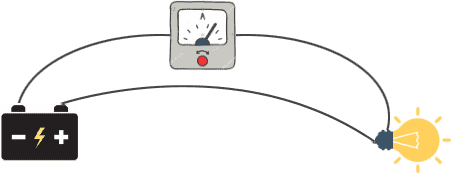Connect a torch bulb and current device to the 4V Battery. The current meter will indicate a current of 0.1 Ampere. The bulb glows and emits light. Here the electric current is converted to light.

#### Case 2: Two Bulbs, Two Batteries, and an Ammeter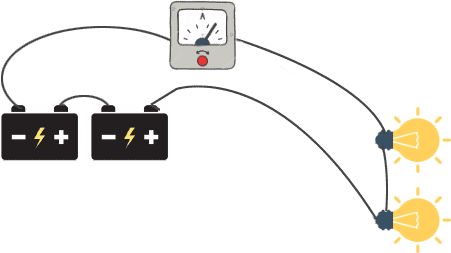Now connect two bulbs and a current meter to 2 Voltage sources. The current drawn is 0.1A and the applied voltage is double than the previous case.

Due to high voltage, the bulbs will glow much brighter. Hence, we can say that power is dependent on the applied voltage.

#### Case 3: Two Bulbs, Single One Battery, and three Ammeters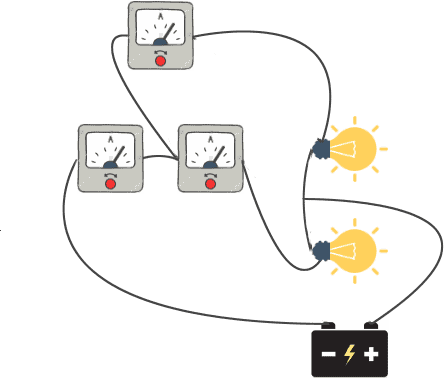Connect the positive lead of the battery to two bulbs along with two ammeters as shown and negative terminal to the third ammeter.

The two bulbs connected to two ammeters show 0.1A and third ammeter shows 0.2A current for the same voltage.

This proves that power is dependent on current as well as voltage.

## Power

You might have noted from the above experiment when an electrical load (bulb) is connected it consumes power.

Electrical Power represented by ‘P’ and it defined as the rate of energy generated or consumed in a circuit.

## Ohms Law Power Relation

Ohms law states current in an electrical circuit is directly proportional to the applied potential and inversely proportional to the resistance.

The formula for the power can be defined in terms of voltage and current.

`Power (W) = Current (I) × Voltage (V) – (Equation 1)`

The units of power are Watt indicated by ‘W’. Semantically I can say, One watt of power is generated if 1 Ampere of current flow through a resistance connected to the 1V battery source.

Since Ohm’s law states that Voltage is the resultant of current multiplied by resistance.

`V = I × R – (Equation 2)`

We can rewrite this equation as I=V/R. If we fix the resistance ‘R’ as constant and increase the voltage (V), the current (I) will be doubled and if we fix the voltage ‘V’ and doubles the resistance the current will become half.

If we replace equation 2 in equation 1 we get,

Power (in terms of current) = I × R × R = I2 R

Similarly, from I=V/R we can get power in the voltage form as

Power (in terms of voltage) = V2/R

If we connect the bulbs for longer duration more amount of energy is consumed and converted into light and heat. Therefore, power is dependent on time also expressed in watt-second.

Formula for power in terms of energy and time is given by

P = W/ t = Work / Time

To keep things simple we can use Ohm’s law circle or triangle to find out the voltage, current, and resistance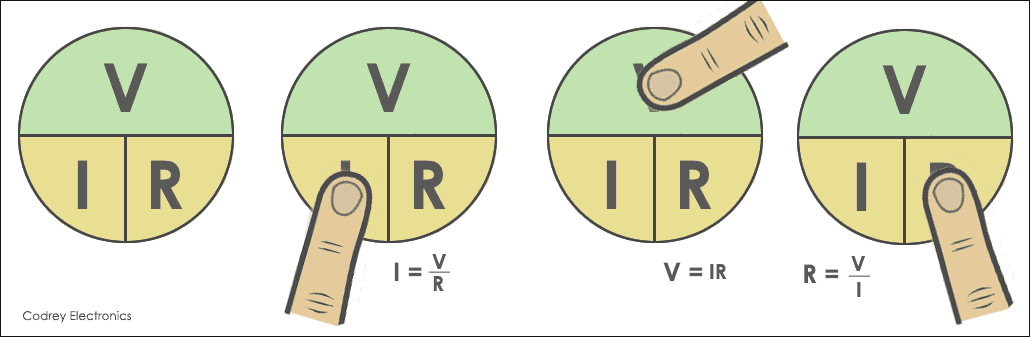## Polarity of Voltage and Current

It is confusing while working with electronic circuits to evaluate voltage and current readings correctly. Proper circuit analysis of current and voltage directions is required to avoid faulty measurements.

## Voltage Direction

The voltage drop in a resistor is from positive to negative direction of conventional current.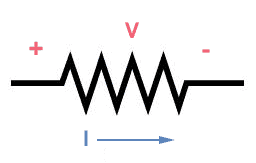## Current Direction

The direction of current plays an important role in understanding power in electric circuits.

In the circuit (a) the current flow from the positive terminal of the battery. This indicates that the current is providing power to the circuit.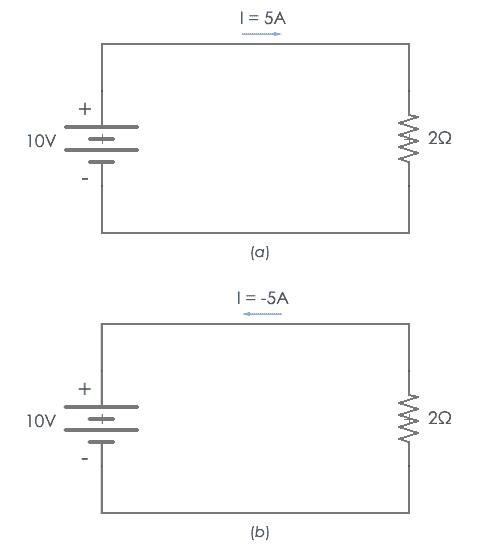Similarly, if the current starts from the negative terminal of the voltage source, it indicates that resistor is consuming power.

## Ohms Law Power Diagram

To understand the ohms law power, Ohm’s Law Circle technique is used in circuit analysis.

The trick used here is, simply multiply or divide the known quantities to get the unknown quantity.

To find out the power (Watts), round of the power, the current and voltage are side by side. So, multiply the current by the voltage.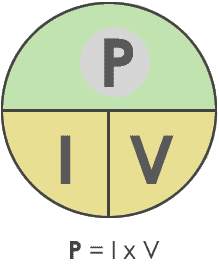Finding Power

To find out the Current (Amperes), round of the current, the power and voltage are diagonal to each other. Hence, divide the Power (Watts) with the voltage (Volts).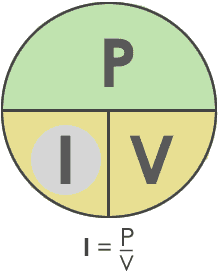Finding Current

Similarly, in order to find out the Voltage (V), round of the voltage and divide the Power (Watts) with the current (Amperes).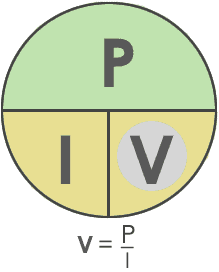Finding Voltage

## Applications of Power

Ohms law is applicable to various appliances around us. Some of them are:

The energy meters used in our homes use kilowatt-hour (KWh) as a practical quantity for measuring energy. Some of the applications of power are:

• Lighting Bulbs in homes and industries
• Heaters
• Motors
• Hair Dryers
• Watt-hour meters
• Electric Vehicles etc.

Conclusion

The electrical load to be connected is dependent upon the power rating of the resistance and wattage. Electronic applications require power dissipation to do their job.

Here power may be positive or negative. The positive power (Bulbs) in electrical circuit indicates the power consumption and negative power (generators) stimulates energy.